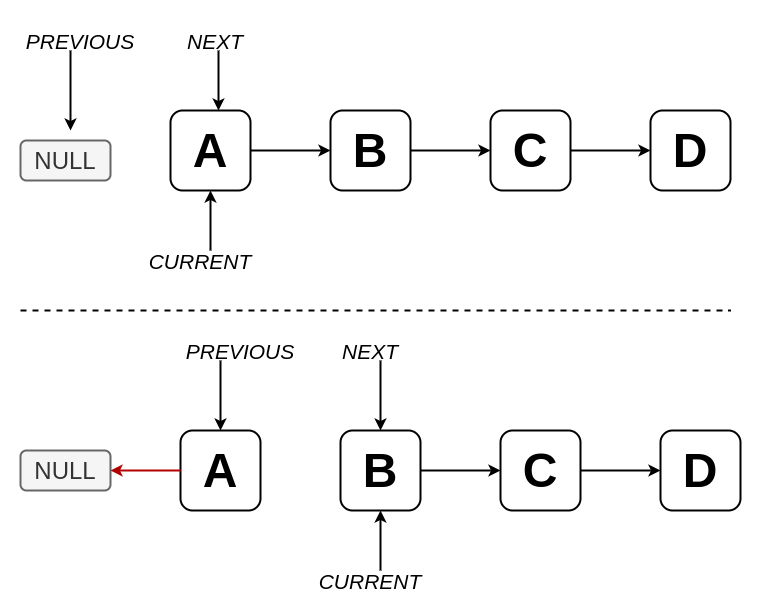# Ricardo Borges

Personal blog## How to reverse a Singly Linked List

In a Singly Linked List, each node has only a pointer to the next node, and there are some ways to reverse it, the one that I want to show here doesn't require extra space.

In this algorithm, we start from the list's head, and we use three pointers, each one for previous node, current node and next node. In the beginning, The current and the next points to the list's head, and the previous points to null. While the current are pointing to a node we do the following:

1. next point to its next node
2. current.next points to previous node
3. previous node points to the current node
4. current node points to next node

This loop continues until the current points to null, which will mean that we reached the end of the list. Like this:

``````1prev = null;
4
5while (current !== null) {
6  next = next.next;
7  current.next = prev;
8  prev = current;
9  current = next;
10}
``````

A single iteration works like this:This algorithm may vary according to the Linked List implementation, using this implementation, looks like this:

``````1function reverse(linkedList: LinkedList<number>) {
2  let prev = null;
5
6  while (current !== null) {
7    next = next.next;
8    current.next = prev;
9    prev = current;
10    current = next;
11  }
12
14}
15
16const linkedList = new LinkedList((a: number, b: number) => a === b);
17